simulation of moving electrons, hydrogen and boron ions in a magnetic fieldA new idea to obtain clean fusion energy

10b Physics experiment: simulation of moving electrons, hydrogen and boron ions in a magnetic field

Computer simulation of idea 10 (and 11, + ).

Fig. 1 The coordinate system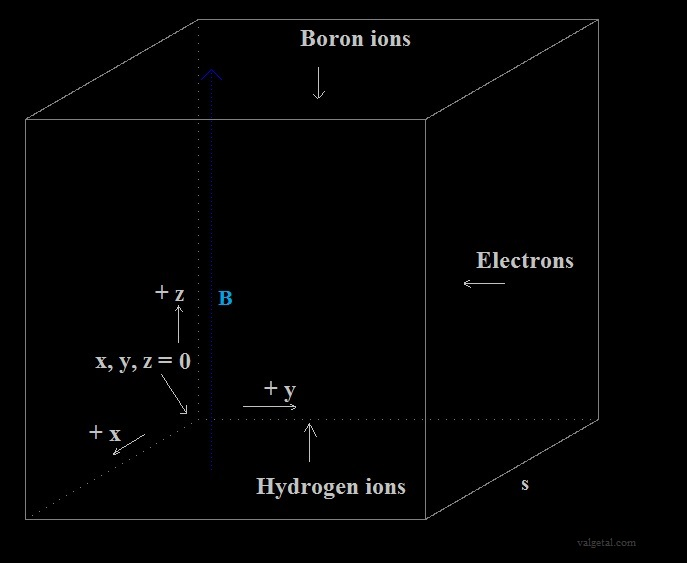The program is programmed in Delphi 4.

We use above displayed  coordinate system.

The sides of the cube are s (s=400 pixels = 1 mtr).
The electrons can be injected in any point (we will do this in such a way that they circle around the centre of the cube).

Actually we could inject any particle with any velocity in any point...

A magnetic field is introduced in the program. A positive value means that the magnetic field B is pointed up and is homogeneous.
(we can B also make variable, for example less in the centre and higher at the sides and near the top and the bottom)

In the program we calculate the electric field and the magnetic field in point (x,y,z) due to a charged moving particle in point (xi,yi,zi) (for non relativistic v<<c)  and adding the fixed magnetic field and electric field.

The electric field in a point (x, y, z):  ( See Coulomb's law )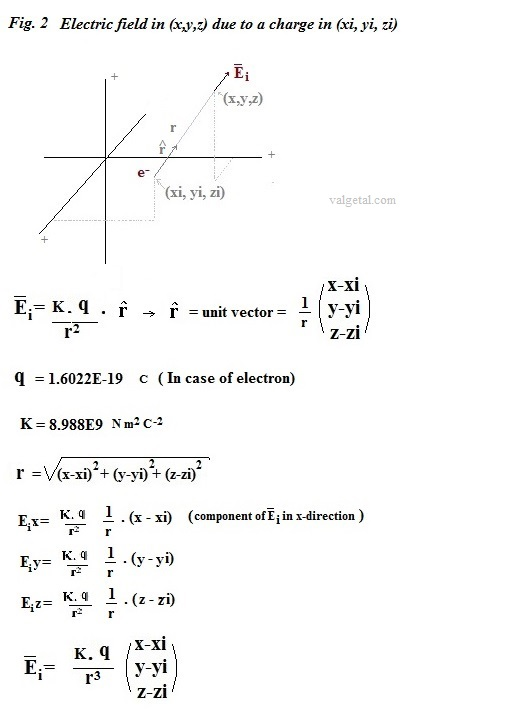The magnetic field we calculate with Biot-Savart Law. (for v<<c)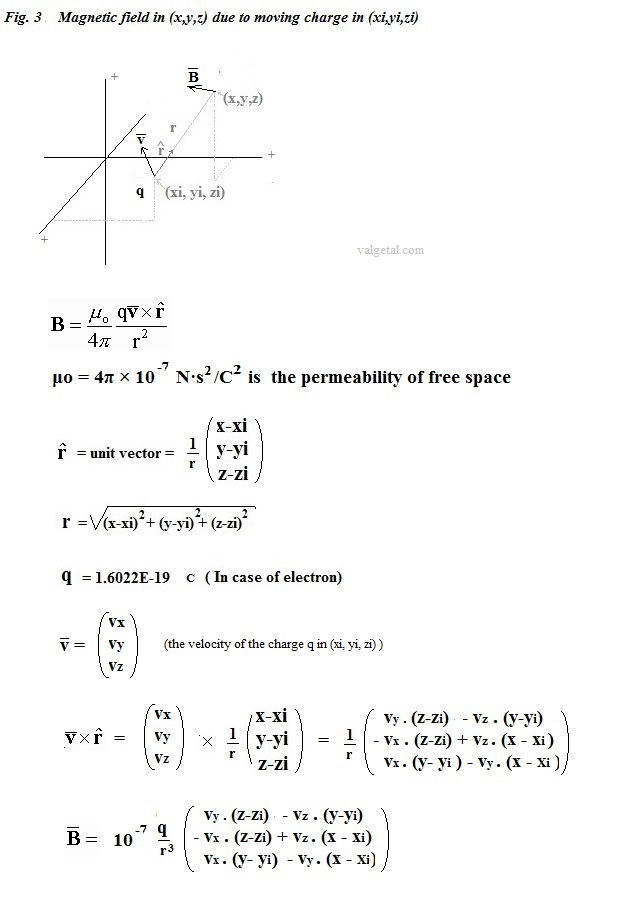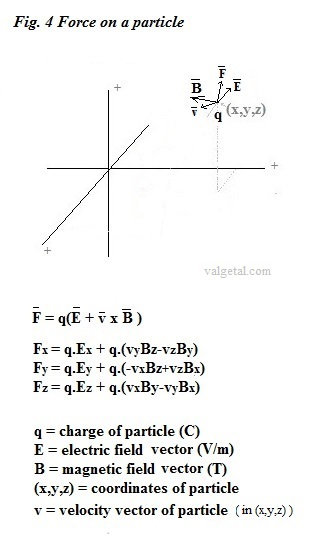Lorentz force

How many electrons produces an electron gun?

A Nanocoulombmeter in combination with a Faraday cup can be used to detect and measure the beams emitted from electron guns and ion guns. (

In the link below an electron gun is sold with an energy range: 1 eV to 100 keV and a beam current range: 1 nA to 20 mA
( )

1.10-9 A = 1.10-9 C/s -> 1.10-9 C/s / (1,6.10-19 C) = 6,3. 109   electrons/s

It seems therefore plausible to have an electron gun that produces about 6.3. 109    electrons/ s
This is  1 electron/1,59 10-10  s

If the energy of an electron is 1 eV, than:

½ meVe2 = 1 eV = 1,602.10-19 J  => Ve = (2 .  1,602.10-19 / (9,1095 . 10-31) ) = 5,931. 10 m/s

---------------

Suppose you have a boron ion with an energy of 4 MeV. What magnetic field is needed to deflect this ion into a circle with a radius of 0,5 mtr?

B= m .v / (r . q )

½ mv2 =  4 .106 . 1,6.10-19 J

=> v = (2 .  4 .106 1,6.10-19 / (11 . 1,67 . 10-27)  )  = 8,35.106  m/s               (boron = 5 proton + 6 neutron,  relativistic effects neglected)

=> B = (11 . 1,67 . 10-27)  . 8,35.106 / (0.5 . 1,6.10-19 ) = 1.9 tesla,  if it's charge is e+    and B = 0,38 tesla if it's charge is 5e+

According en.wikipedia.org/wiki/Orders_of_magnitude_(magnetic_field) this (2 T)  is a magnetic field generated in a modern 60 Hz power transformer.

The remanent field of a neodymium magnet is typically about 1-1.4 tesla,  see en.wikipedia.org/wiki/Neodymium_magnets

To construct a electromagnet of 2 Tesla seems to be a bit difficult, see  instructables.com/answers/2-Tesla-Electromagnet/

https://en.wikipedia.org/wiki/Particle-in-cell

---------------

Experiments with the program:

 If r < (1E-8) then avoid calculate the field of a particle in (x,y,z), because r=0 end field too big Experiment 1: 10 Boron+ ions started with speed=0 above at (x=0,5s, y=0,5s, z=0,75s) and 10 H+ ions started with speed=0 below at (x=0,5s, y=0,5s, z=0,25s), see fig. 1 for coordinate system. Click in the image for a full screenshot. First the electrons moved together in a circle. Later on they separate in three different circles. Also curious is that a few H+ and B+ ions obtain more kinetic energy because they move more up and down (farther away) than there initial positions. It seemed to be that they collided amongst themselves. Curious, because the possibility of colision was not programmed.''                     The same experiment, but more time elapsed: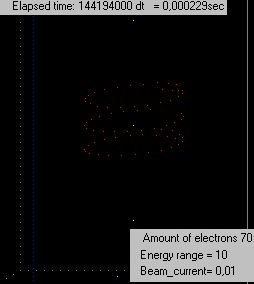Experiment 2:  3 Boron+ ions started with speed=0 above at (x=0,5s, y=0,5s, z=0,75s) and 3 H+ ions started with speed=0 below at (x=0,5s, y=0,5s, z=0,25s). The electrons were obtaining more speed (kinetic energy); in some configurations (B-field, initial speed etc.) they quite rapidly increased their radius. So a new procedure was introduced in the program: if (the total kinetic energy of all electrons ) - (initial kinetic energy of all electrons) > 10-14  J , then the speed of all electrons is decreased untill the difference <10-14 J Experiment 3: 3 Boron+ ions started with speed=0 above at (x=0,5s, y=0,5s, z=0,75s) and 3 H+ ions started with speed=0 below at (x=0,5s, y=0,5s, z=0,25s).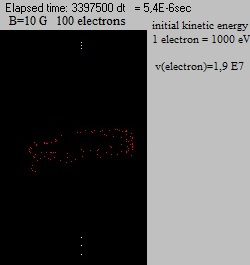The electrons start to spread upwards and downwards. It´s a pity, but they do not keep nicely moving in a circle.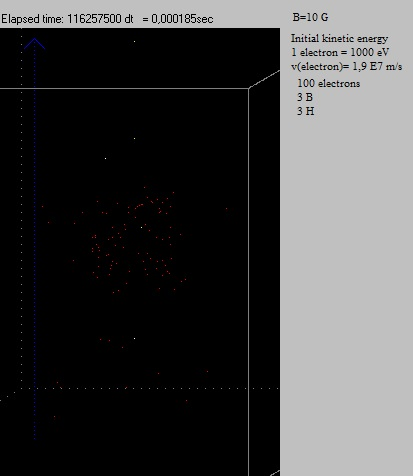The same experiment, but a few days later. The electrons did spread even more, although still moving in circles around the z-axis. Experiment 4: 3 Boron+ ions started with speed=0 above at (x=0,5s, y=0,5s, z=0,75s) and 3 H+ ions started with speed=0 below at (x=0,5s, y=0,5s, z=0,25s).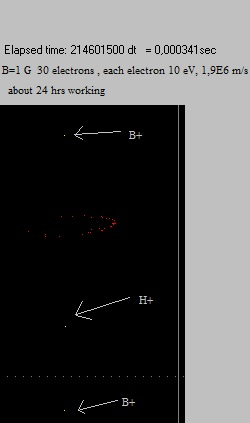One B+ and 1 H+ have disappeared (out of screen), probably because collision and obtaining a high speed. Also curious there has appeared a gap in the ring with electrons. Experiment 5: Instead of electrons, 20  Boron+ ions, circulating with the clock. The B field is first 10000 G, and then increased to 16000 G. Experiment 6: 30 electrons, 100 eV, 0,001 nA, 3 B+, 3 H+, B=10 G, after about 15 min (just generated all 30 electrons), increased the field to B=100 G, resulting in a kind of "pulsating circle" of electrons, which seems to be not spreading out... More about experiment 6 (and 7) Experiment 7: 50 electrons, 100 eV, 0,001 nA, 3 B+, 3 H+, B=10 G, after about 30 min (just generated all 50 electrons), increased the field to B=100 G  In reality it will not be possible to change the magnetic field instantaneously, because, if we use an electromagnet, it will have an selfinductance which will delay the building up of a new magnetic field.. Therefore we simulate this by distributing the electrons randomly inside a circle determined by the old and the new B-field (see More about experiment 6 &7  )Video experiment 7   (after 5,18E-5 s) After 5,18E-5 sec, about 8 hrs computer time, the electrons still did not spread out.. Video experiment 7 (after 0.000173 s) And after 0,000173 s neither....  (remark: in the program the total kinetic energy of all electrons is checked all the time, and stays constant; when it increases to much, the speed of each electron is multiplied with a factor 0,999 untill it´s again within limits) Video experiment 7 (after 0.00021 s) Video experiment 7 (after 0.00036 s) A few electrons are now a bit farther away from the centre. Video experiment 7 (after 0.00037 s)  They came again a little bit closer to the centre. Video experiment 7 (after 0.000596 s)  1 or 2 electrons have escaped out of the chamber. The rest are still ubicated in the centre, but seemed to have slowed down and moving in small circles. In the program the total kinetic energy of the electrons cannot increase. If it increases a little bit  then the speed of each electron is multiplied with 0.999 in a repeat loop, until the present total kinetic energy of the electrons < initial total kinetic energy. But the total kinetic energy can yes decrease, and that is probable what has happened (due to the fact the this simulation is an approximation of reality, the same way you can approximate a smooth line by a stepwise drawn line... Experiment 8: In the former experiments we generated the electrons in a point of their orbiting circle inside the vacuum chamber. In reality not very handy to let the electron gun point inwards the vacuum chamber were fusion should take place. Let´s now see if it is possible to generated them in point (0,5s, s, 0,5s), in the middle of the right side, and let them again orbit in a circle around the centre of the cube... Experiment 9:From experiment 3 the total kinetic energy of all particles can not increase (when it increases beyond a limit, the speed of the electrons is reduced a bit until it´s again under this limit). The kinetic energy can however yes decrease, and this is what possible happened in experiment 7. Now in experiment 9 it can neither decrease. Furthermore the potential energy is calculated . Experiment 10:The simulation program has been refined by implementing the Leapfrog intergration method. Only  positive ions (H+, B+)  are released in the vacuum chamber (no electrons): above and under positive point charges are placed and a vertical magnetic field is applied. The goal is to confine the positive ions.

 Some wild ideas First idea (Jan. 2013) (Jan. 2013) (Jan. 2013) (3 Febr. 2013) (6 Febr. 2013) (8 Febr. 2013) (16 Jan. 2014) (17 Jan. 2014) (20 Jan. 2014) ( Febr. 2014- 2016) (not working)

Computer simulation tenth idea (2014-2016)
Eleventh idea   (2016.. )

20 February 2014 -.     by  Rinze Joustra        www.valgetal.com